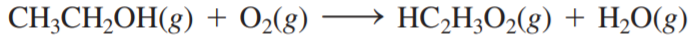×
Get Full Access to Chemistry: A Molecular Approach - 3 Edition - Chapter 18 - Problem 86e
Get Full Access to Chemistry: A Molecular Approach - 3 Edition - Chapter 18 - Problem 86e

×

# Refer to the tabulated values ofISBN: 9780321809247 1

## Solution for problem 86E Chapter 18

Chemistry: A Molecular Approach | 3rd Edition

• Textbook Solutions
• 2901 Step-by-step solutions solved by professors and subject experts
• Get 24/7 help from StudySoup virtual teaching assistantsChemistry: A Molecular Approach | 3rd Edition

4 5 1 263 Reviews
25
3
Problem 86E

Refer to the tabulated values ofin Appendix IIB to calculatefor the fuel cell breathalyzer, which employs the following reaction. (for HC2H3O2(g) = -374.2 kJ/mol.)Step-by-Step Solution:
Step 1 of 3

Effective nuclear charge Explanation of many atomic properties (ionization energy etc.) relies in part on understanding how effective nuclear charge varies throughout the periodic table – there are several ways of calculating Z eff – simple empirical guides, such as Slater’s rules, can provide insight Slater’s rules ▯ Used to estimate effective nuclear charges ▯ [1s][2s2p][3s3p][3d][4s4p][4d][4f][5s5p][5d][5f]... ▯ All electrons to the right contribute 0 to shielding ▯ All electrons in the same group contribute 0.35 to shielding ▯ For s and p electrons, all electrons in the (n-1) shell contribute 0.85 each to the shielding ▯ All other electrons to the left contribute 1.0 to the shielding ▯ When considering [nd] or [nf] electrons, all electrons to the left contribute 1.0 to shielding Periodic variation of Z eff Increases left to right across a row Roughly constant down a group after an initial increase

Step 2 of 3

Step 3 of 3

##### ISBN: 9780321809247

Chemistry: A Molecular Approach was written by and is associated to the ISBN: 9780321809247. This full solution covers the following key subjects: refer, tabulated, values. This expansive textbook survival guide covers 82 chapters, and 9454 solutions. This textbook survival guide was created for the textbook: Chemistry: A Molecular Approach, edition: 3. The full step-by-step solution to problem: 86E from chapter: 18 was answered by , our top Chemistry solution expert on 02/22/17, 04:35PM. Since the solution to 86E from 18 chapter was answered, more than 285 students have viewed the full step-by-step answer. The answer to “?Refer to the tabulated values of in Appendix IIB to calculate for the fuel cell breathalyzer, which employs the following reaction. ( for HC2H3O2(g) = -374.2 kJ/mol.)” is broken down into a number of easy to follow steps, and 27 words.

Unlock Textbook Solution